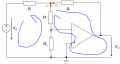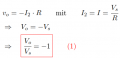# Ideal OP-AMP: Resistance parallel to internal connection between + and - input

#### koteles

Joined Jul 10, 2019
4
Hi,

I'm stuck trying to find and explanation for a given solution to an OP-Amp exercise:The goal is simply to find an expression that connects the output V_O to the input V_S.

This is the solution provided:Is that solution correct? There's no explanation to it and the only way I can make sense of it is this:
• No current can flow through the I_1 branch, because
• R_x is in parallel to the inputs of the OP-Amp
• In the ideal case, the inputs have the same voltage
• Therefore no voltage can drop over R_X, so no current can flow there either
• Since no current can flow through the inputs of the OP-Amp, the entire I_1 branch can't have current and is therefore irrelevant
• Current can flow through the I_2 branch
• (I guess from the end of that branch to the +-Input of the OP-Amp?)
With these assumptions, the two mesh equations around the blue circles I drew yield this result. Is this (and all my assumptions above) correct? I also got the exact same final result with a completely different (contradictionary) approach. If this one isn't correct I'll describe the other one.

Thanks a lot

#### WBahn

Joined Mar 31, 2012
27,857
Hi,

I'm stuck trying to find and explanation for a given solution to an OP-Amp exercise:

View attachment 181431

The goal is simply to find an expression that connects the output V_O to the input V_S.

This is the solution provided:
View attachment 181430

Is that solution correct? There's no explanation to it and the only way I can make sense of it is this:
• No current can flow through the I_1 branch, because
• R_x is in parallel to the inputs of the OP-Amp
• In the ideal case, the inputs have the same voltage
• Therefore no voltage can drop over R_X, so no current can flow there either
• Since no current can flow through the inputs of the OP-Amp, the entire I_1 branch can't have current and is therefore irrelevant
• Current can flow through the I_2 branch
• (I guess from the end of that branch to the +-Input of the OP-Amp?)
With these assumptions, the two mesh equations around the blue circles I drew yield this result. Is this (and all my assumptions above) correct? I also got the exact same final result with a completely different (contradictionary) approach. If this one isn't correct I'll describe the other one.

Thanks a lot
Your reasoning is correct except for the claim that the I1 branch is irrelevant -- remove it and your circuit won't work.

But, in the case of an ideal opamp, the values of the resistors in that branch are irrelevant. Rx could be removed completely and the R in it could be replaced with a short.

When you start working with non-ideal opamp circuits, you will start to see why these resistors are there and how to properly size Rx.

Joined Mar 10, 2018
4,057
To further WBahn comments, the case we are discussing is DC. The OpAmp
has finite G (Aol, open loop gain). Aggravating this is as frequency rises the OpAmp
gain drops. What looks like hi Z inputs, low z output, of the OpAmp, becomes
compromised as freq rises as it runs out of G.Regards, Dana.

#### koteles

Joined Jul 10, 2019
4
Perfect, thanks for the effort! So can I always deduct that, if the voltage is (forced to be) the same at two ends of a resistor, like it's the case for R_X here, no current can flow there either? Makes sense mathematically, I just want to make sure it's always correct for similar problems.

Could you also tell me, why current can flow through the I_2 branch? Does it indeed go into the + sign (unaltered by "internals" of the OP-Amp), while both ends still don't have the same voltage?

#### WBahn

Joined Mar 31, 2012
27,857
Perfect, thanks for the effort! So can I always deduct that, if the voltage is (forced to be) the same at two ends of a resistor, like it's the case for R_X here, no current can flow there either? Makes sense mathematically, I just want to make sure it's always correct for similar problems.
If a resistor has no voltage across it, then it has no current through it and vice-versa (unless we are talking about a superconductor or about noise-level signals).

Could you also tell me, why current can flow through the I_2 branch? Does it indeed go into the + sign (unaltered by "internals" of the OP-Amp), while both ends still don't have the same voltage?
Huh?

I2 is the current flowing in the feedback resistor. What "+ sign" are you talking about?

The opamp will output whatever voltage is needed (and source/sink whatever current is needed) in order to make the voltage difference between its inputs effectively zero. In this circuit, that means taking the output sufficiently negative so that all of the current going through the left resistor from the signal source goes through the feedback resistor while making the voltage at the junction of the two equal to zero.

#### koteles

Joined Jul 10, 2019
4
Thanks again. With the second question I basically just wanted to know what happens to the current of the I_2 branch at the output of the OP-Amp and if I need to know that in some other cases where I might want to find a loop equation for it. I realized now that "flowing into the +" doesn't make any sense as the current flow is zero there anyway.

In the non-ideal model the output is connected to the ground through a voltage source and a resistor. Can I still use this "connection" in the ideal case to create a loop equation? I was initially confused about this and though that no current can flow through I_2 at all since V_O is not connected and the internals of the OP-Amp are usually disregarded in the ideal case, which is why there is no visible connection to anything for me and I couldn't image how/where current could flow.

Joined Mar 10, 2018
4,057
All OpAmps have a bias current into/out of their inputs. But todays OpAmp,
voltage OpAmps, have really low current that can be ignored except in very
high impedance environments, like charge amplifier, electrometers, where
very low currents can cause large offsets in very high impedance applications.
Applications where minute dust and films on boards cause issues.

Think Meg to Gig Ohms.....

Regards, Dana.

#### WBahn

Joined Mar 31, 2012
27,857
Thanks again. With the second question I basically just wanted to know what happens to the current of the I_2 branch at the output of the OP-Amp and if I need to know that in some other cases where I might want to find a loop equation for it. I realized now that "flowing into the +" doesn't make any sense as the current flow is zero there anyway.

In the non-ideal model the output is connected to the ground through a voltage source and a resistor. Can I still use this "connection" in the ideal case to create a loop equation? I was initially confused about this and though that no current can flow through I_2 at all since V_O is not connected and the internals of the OP-Amp are usually disregarded in the ideal case, which is why there is no visible connection to anything for me and I couldn't image how/where current could flow.
Disregarding the internals of the opamp means ignoring the non-ideal behaviors of it. We treat the output as an ideal voltage source, meaning that it can source/sink whatever current is needed in order to establish the programmed voltage at the output.